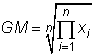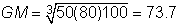### Tip of the Week #104                   Tip Index

Go to the Prior Tip Trading Chaos: Applying Expert Techniques to Maximize your Profits
Go to the Next Tip  The Paradox of Choice by Barry Schwartz

# Geometric Mean and Harmonic Mean

A statistic is simply a number that describes something about a population (i.e., probability density function) or data.  Mainly, statistics describe where the distribution is located or something about  its shape.

One class of statistics is central measures which describe where a distribution is located or "centered" on the x-axis.  These are often considered "averages," though the appropriate average depends upon the use.

The most popular central measures are

• mean (the probability-weighted average of a population or an average of data samples)
• median (the middle value)
• mode (the most probable value)

By far the most useful of these is the mean.  The median and mode are essentially worthless in economic evaluation.  I use the mode only on occasions when describing a triangle distribution.

There are many additional central measures that are better-suited to particular uses.  One source (wikipedia.org) lists 23 central measures!  Not to worry: the mean is the appropriate single-point forecast 95+% of evaluation uses. Following are two additional "averages" that you may find interesting and useful.

## Harmonic Mean

The harmonic mean is a better "average" when the numbers are defined in relation to some unit.  The common example is averaging speed.

For example, suppose that you have four 10 km segments to your automobile trip.  You drive your car:

• 100 km/hr for the first 10 km
• 110 km/hr for the second 10 km
• 90 km/hr for the third 10 km
• 120 km/hr for the fourth 10 km.

 Distance Velocity Time km km/hr hr 10 100 0.100 10 110 0.091 10 90 0.111 10 120 0.083 40 0.385 103.80 Avg V

The harmonic mean formula is:Excel calculates this with the formula   =HARMEAN(100,110,90,120).  Unfortunately, the formula is not generalized to average velocities if across different distances.

In petroleum engineering, the harmonic mean is sometimes the better "average" for vertical permeability with horizontally-layered bedding.

## Geometric Mean

In economic evaluation work, the geometric mean is often useful.The large P represents multiplication, analogous to the S representing summation.

The GM is for situations we want the average used in a multiplicative situation, such as the "average" dimension of a box that would have the same volume as length x width x height.  For example, suppose a box has dimensions 50 x 80 x 100 cm.A cubic box with sides of the GM=73.7 cm would enclose the same 400,000 cm3 or 0.4 m3  volume.

The customary economic evaluation application is in determining "average" inflation or rate of return across several time periods.  In calculating the GM, the numbers must all be positive.  So, add 1 to each value before calculating the GM and subtract 1 from the answer.

Suppose that your portfolio has these five annual returns: .10, -.20, 0, -.10, .20.  The order does not matter if the portfolio has no contributions or withdrawals during the five years.  The return arithmetic average is 0.  However, a portfolio across five years with these annual returns would lose about 5% of value.  The geometric mean:In checking:  (1.1)(0.8)(1.0)(0.9)(1.2) = 0.95 = (1-.010)5.  That is, a portfolio with this a GM = -.01 would lose .05 in five years.

In Excel the answer is calculated with the formula  =GEOMEAN(1.1,.8,1,.9,1.2)-1

Beware when someone says he used an "average" value in a calculation.  Any of the central measures means "average" in some context.  In economic evaluation, the appropriate single-point forecast is almost always the mean. (which is a sample mean or expected value, depending upon whether the distribution represents data or judgment).  For inflation, price escalations, and rates of return, the geometric mean is the appropriate single-point representing an average across time periods.

—John Schuyler, January 2005.